Homework Help Question & Answers

# 3) At the instant shown, car A has a constant velocity of 90 km/h, and car...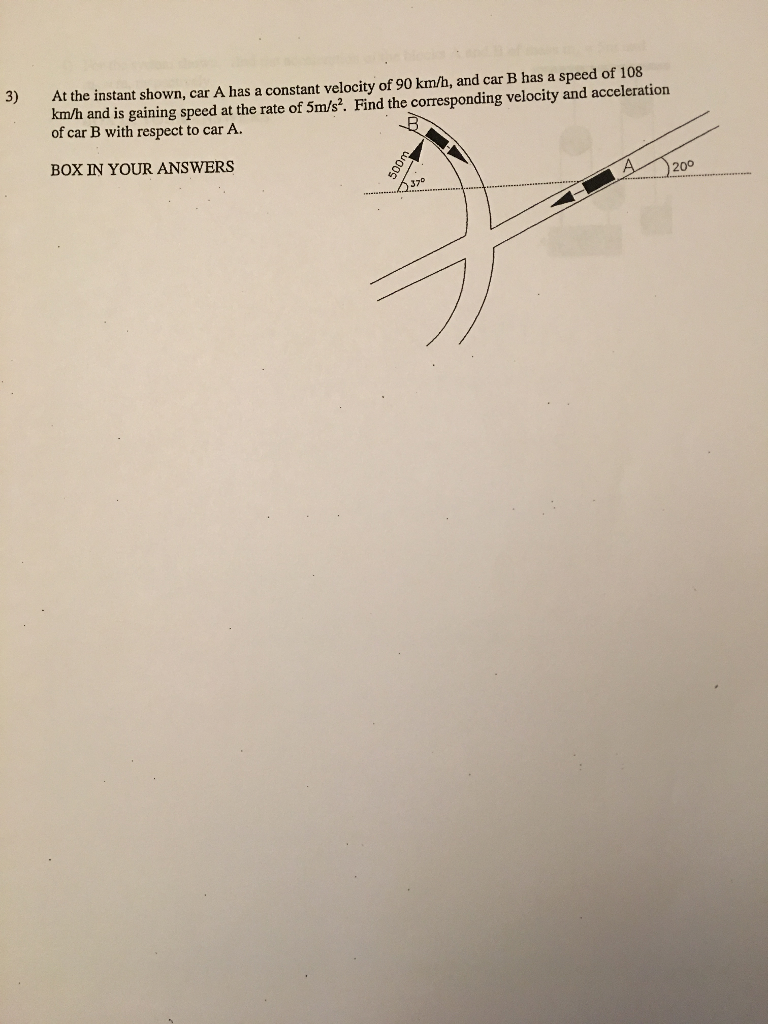3) At the instant shown, car A has a constant velocity of 90 km/h, and car B has a speed of 108 km/h and is gaining speed at the rate of 5m/s2. Find the corresponding velocity and acceleration of car B with respect to car A. BOX IN YOUR ANSWERS 20° 37D

#### Homework Answers

Answer #1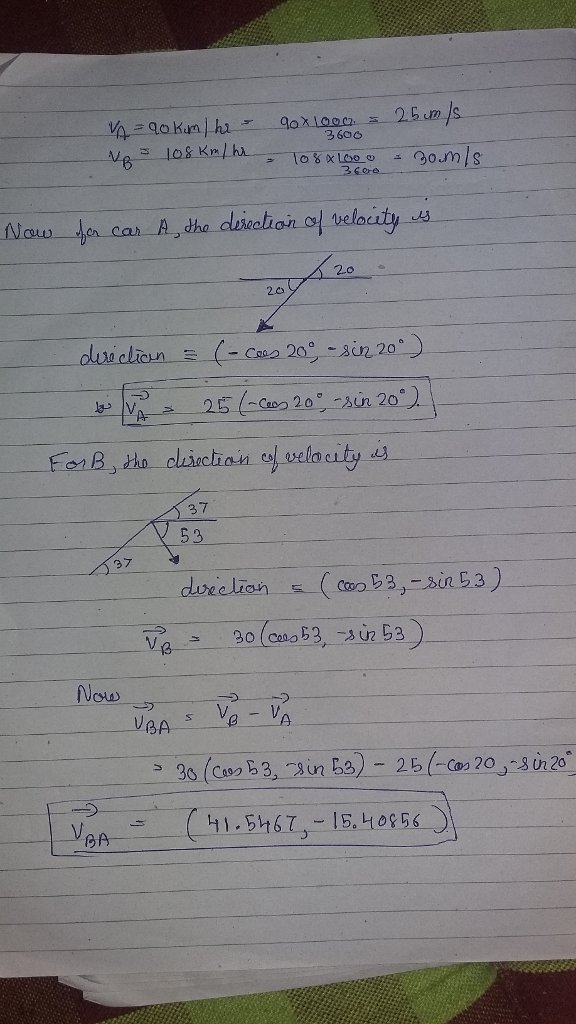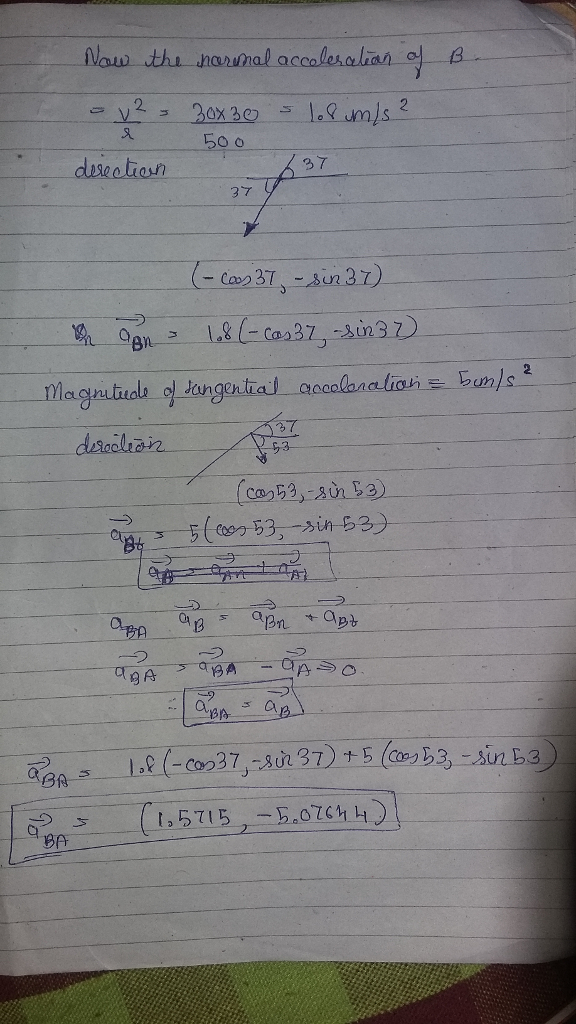Know the answer?
Your Answer:

#### Post as a guest

Your Name:

What's your source?

#### Earn Coin

Coins can be redeemed for fabulous gifts.

Not the answer you're looking for? Ask your own homework help question. Our experts will answer your question WITHIN MINUTES for Free.
Similar Homework Help Questions
• ### Car A is traveling at the constant speed of 61 km/h as it rounds the circular...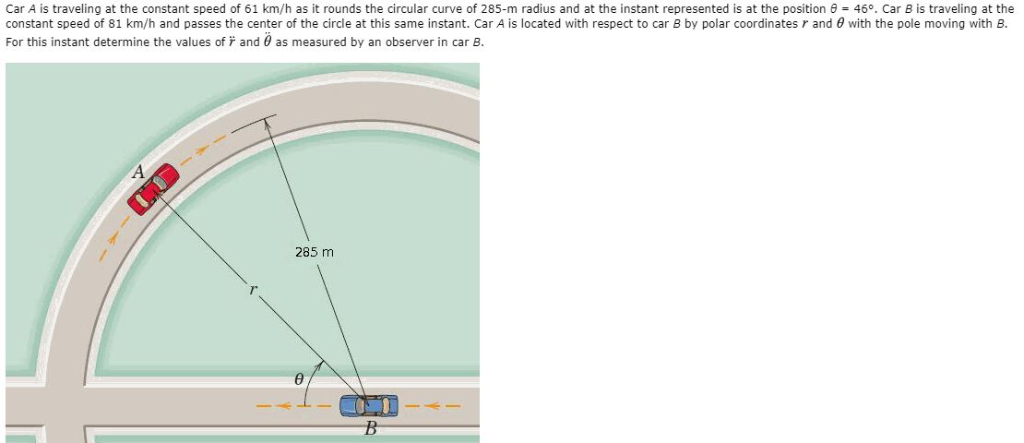Car A is traveling at the constant speed of 61 km/h as it rounds the circular curve of 285-m radius and at the instant represented is at the position 8 46°. Car B is traveling at the constant speed of 81 km/h and passes the center of the circle at this same instant. Car A is located with respect to car B by polar coordinates r and with the pole moving with B For this instant determine the values of...

• ### The car is travelling at a speed of 100 km/h and as it decelerates at 2...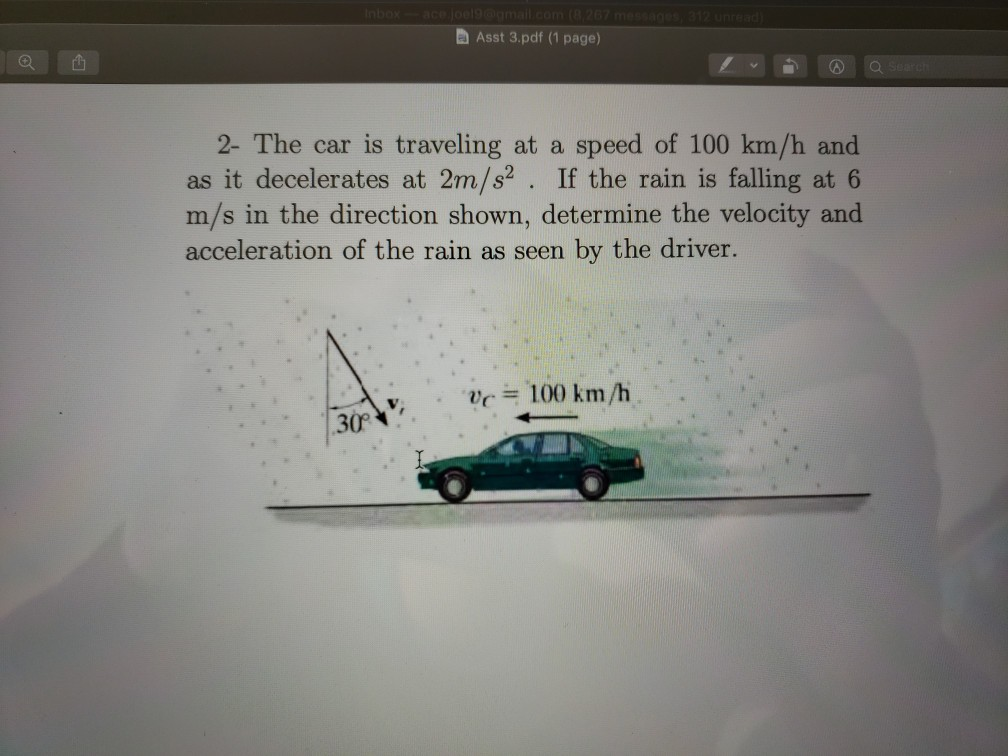The car is travelling at a speed of 100 km/h and as it decelerates at 2 m/s^2. If the rain is falling at 6 m/s in the direction shown, determine the velocity and acceleration of the rain as seen by the driver. Asst 3.pdf (1 page) 2- The car is traveling at a speed of 100 km/h and as it decelerates at 2m/s2. If the rain is falling at 6 m/s in the direction shown, determine the velocity and acceleration...

• ### Dynamics Question! At the instant illustrated, car 8 has a speed of 27 km/h and car...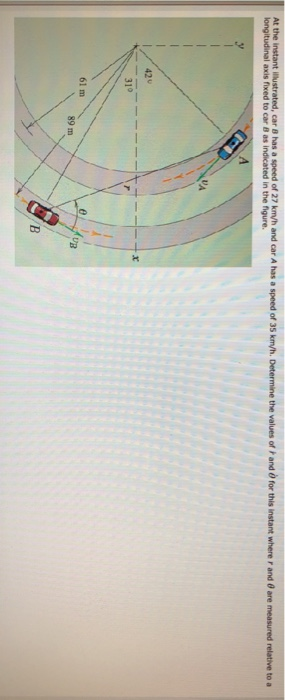Dynamics Question! At the instant illustrated, car 8 has a speed of 27 km/h and car A has a speed of 35 km/h. longitudinal axis fixed to car B as indicated in the figure. relative to a 42U 310 61 m 89 m

• ### Knowing that at the instant shown crank BC has a constant angular velocity of 47 rpm...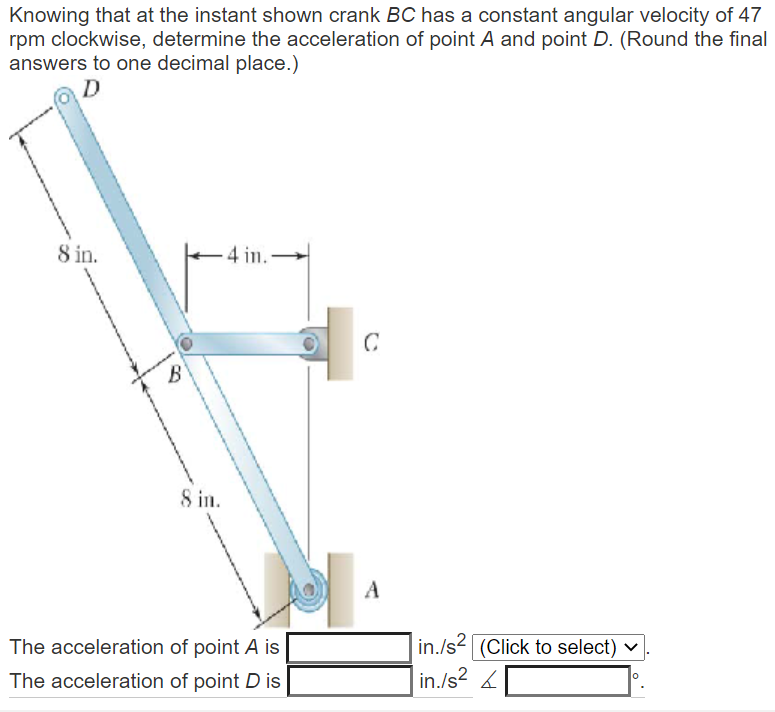Knowing that at the instant shown crank BC has a constant angular velocity of 47 rpm clockwise, determine the acceleration of point A and point D. (Round the final answers to one decimal place.) D 8 in. - 4 in с B 8 in. А The acceleration of point A is The acceleration of point D is in./s2 (Click to select) v in./s2 <

• ### (25 points) At the instant shown, car A is rounding a curve at a speed of...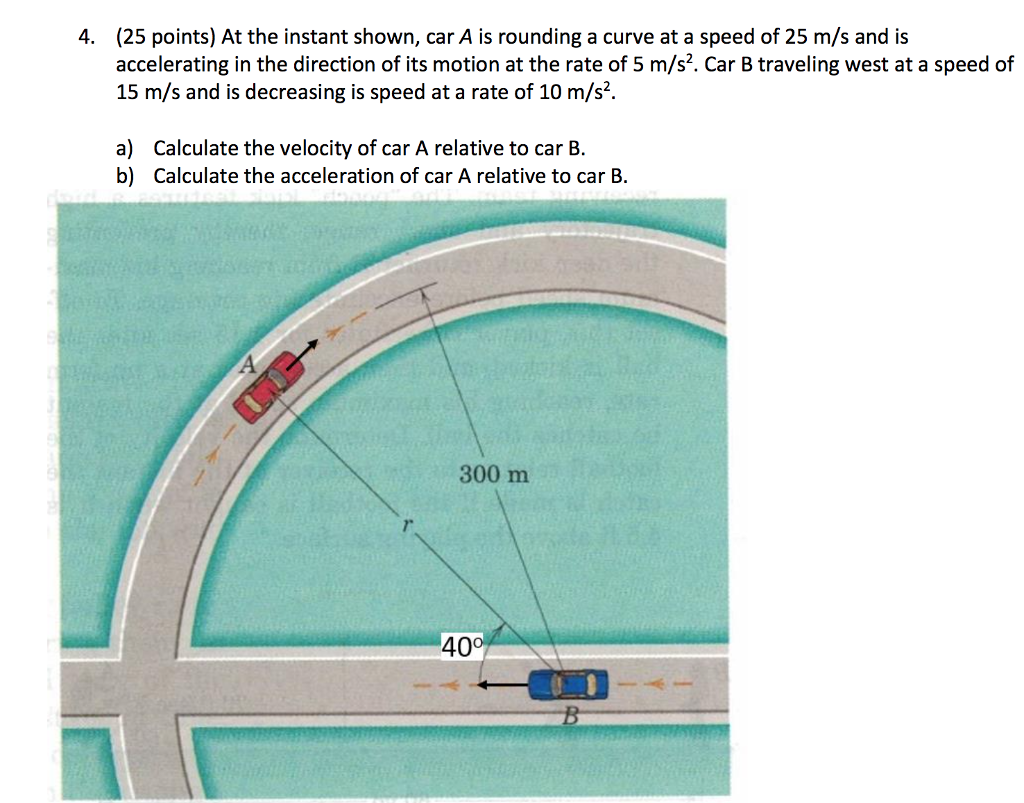(25 points) At the instant shown, car A is rounding a curve at a speed of 25 m/s and is accelerating in the direction of its motion at the rate of 5 m/s2. Car B traveling west at a speed of 15 m/s and is decreasing is speed at a rate of 10 m/s2 4. a) Calculate the velocity of car A relative to car B. Calculate the acceleration of car A relative to car B. b) 300 m 7....

• ### P6 A car travels around a circular track at constant speed, as shown. It is observed...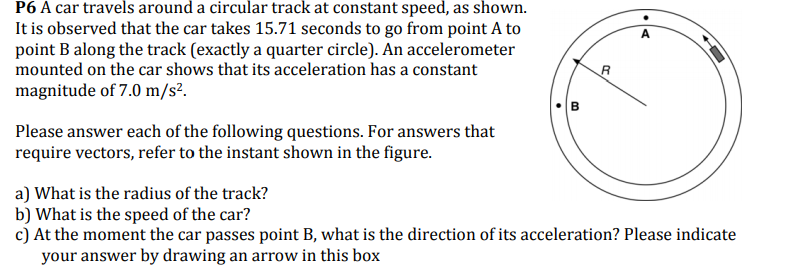P6 A car travels around a circular track at constant speed, as shown. It is observed that the car takes 15.71 seconds to go from point A to point B along the track (exactly a quarter circle). An accelerometer mounted on the car shows that its acceleration has a constant magnitude of 7.0 m/s2. Please answer each of the following questions. For answers that require vectors, refer to the instant shown in the figure. a) What is the radius of...

• ### P6 A car travels around a circular track at constant speed, as shown. It is observed...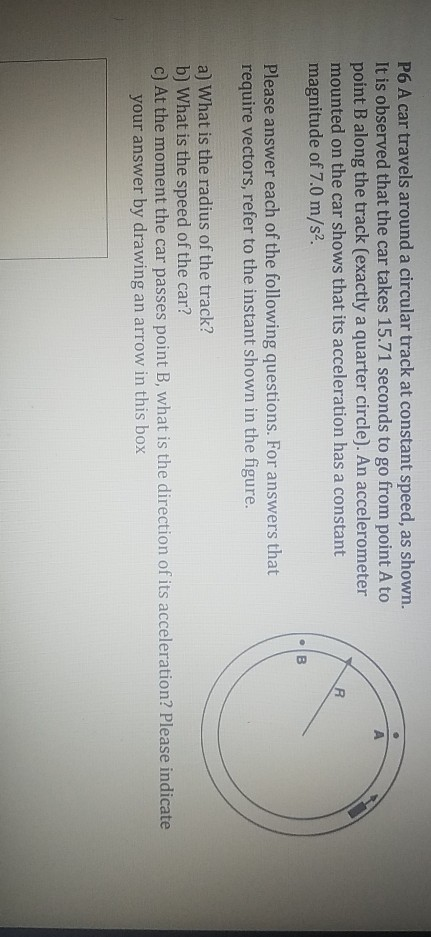P6 A car travels around a circular track at constant speed, as shown. It is observed that the car takes 15.71 seconds to go from point A to point B along the track (exactly a quarter circle). An accelerometer mounted on the car shows that its acceleration has a constant magnitude of 7.0 m/s2. Please answer each of the following questions. For answers that require vectors, refer to the instant shown in the figure. a) What is the radius of...

• ### Two highways intersect as shown in the figure. At the instant shown, a police car s...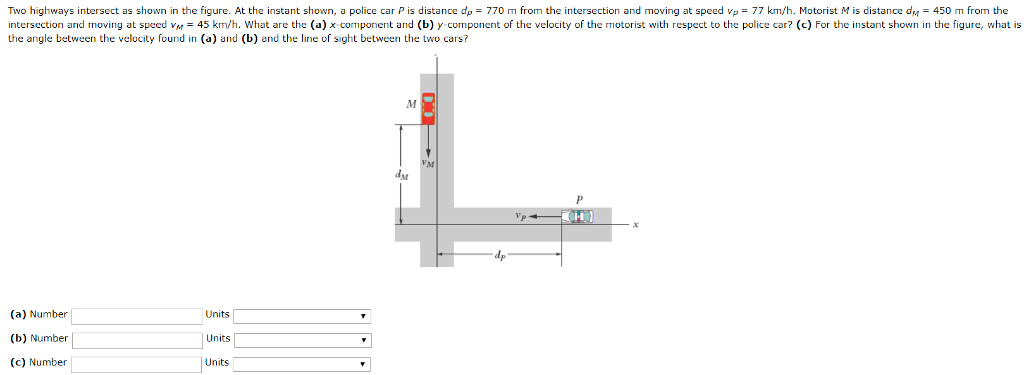Two highways intersect as shown in the figure. At the instant shown, a police car s distance d p 770 m rom the intersection and moving at speed vp = Motorist M is distance dM 450 m rom the intersection and moving at speed vM45 krn/h. What are the (a) x component and (b) y component of the velocity of the motorist with respect to the police car? (c) For the instant shown in the figure, what is the angle...

• ### A car travels at a constant velocity of 90 km/h along an expressway under-ambient temperature and...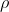A car travels at a constant velocity of 90 km/h along an expressway under-ambient temperature and pressure conditions. The width of the car is 1.5 m while its height is 1.2 m. The wheelbase (length between the front and rear wheels) is 2.5 m. The drag coefficient is 0.22. [Use air, ambient density of the air = 1.12 kg/m3 and, fuel, density of the fuel = 930 kg/m3. You may neglect any energy contained in the exhaust gases and any...

• ### to The car A has a forward speed of 21 km/h and is accelerating at 2.5...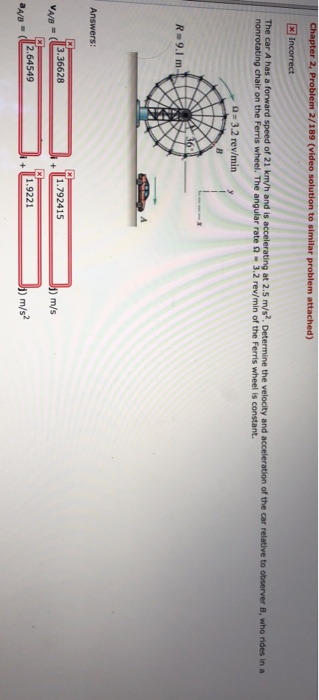to The car A has a forward speed of 21 km/h and is accelerating at 2.5 m/s?. Determine the velocity and nonrotating chair on the Ferris wheel. The angular rate Ω-3.2 rev/min of the Ferris wheel is constant. n of the car relative to observer B, who rides in a a- 3.2 rev/miny R-9.1m Answers un·13.36628 1.792415 m/s aad 2.64549 1.9221 ) m/s2

Free Homework App

Scan Your Homework
to Get Instant Free Answers
Need Online Homework Help?

Get Answers For Free
Most questions answered within 3 hours.